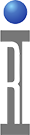For searches such as P1dB and Bias Values, using the "find index of b in a" and "value at index b" System CALC buttons

Two calculation buttons have been created that provide a powerful tool to use in dependent value searches. These include P1dB and specific bias conditions such as finding a base voltage that produces a drain current. The basic concept involves measuring the dependent and independent values, placing them in indexed arrays, finding the index that gives the desired condition in the dependent array, then using that index to extract the independent variable that creates the desired dependent condition: This algorithm expects data that consistently trends up or down. If the relation ship between the first and last value in the array varies, the direction of search will vary.

In the test plan we need to:

1.) Collect two arrays of data that share a common index,

2.) Establish what the desired dependent condition is:

3.) Use the "find index of B in A" button
a.) This fits a curve to the "A" (dependent) array. (Allows us to get the exact value between the measured points)
b.) Extracts the index of the desired value ("B") in the "A" array.

4.) Then Use the "value at index B" button
a.) Input the independent array into the "A" side of the button.
b.) Input the selected index from above into the "B" side.

5.) This outputs the independent value that gives the dependent condition.

In the specific case of P1dB we need to:

1.) Measure the small signal gain, in this case 10dB

2.) Subtract 1 from the value to establish the "target compression gain" in the=is case 9dB

3.) Measure gain at a number of Source 1 input power levels, saving the measured gains, indexed by Source 1 Power (The index value is actually not the Source 1 power value, It is the "N" as in the Nth value of the Source 1 power used).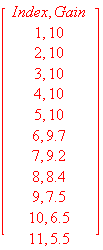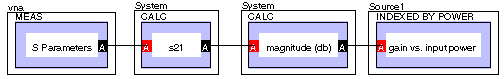4.) In addtion we must save the second array which is the actual Source 1 power, also indexed by Source 1 Power.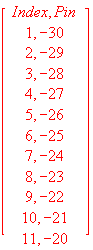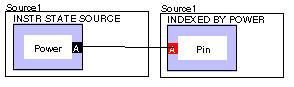5.) Now that the arrays are full, we need to find the fractional index for the 1 dB compressed gain value (9 dB in this case). This would be an index value of approximately 7.2. This is the "find index of b in a" button.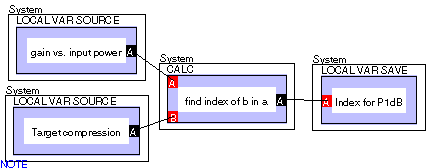6.) Now we need to extract the Pin that corresponds to that index. This would be a Pin of approx -23.8dBm This is the "value at index b" button.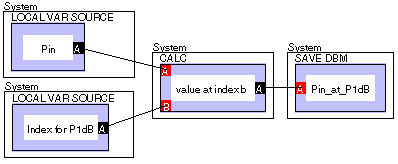7.) Of course this can be combined into one panel.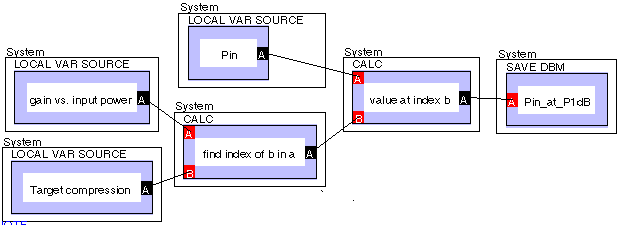Attached is a version of the test plan: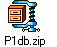https://roos.com/docs/RBEH-4MER9T
ROOS INSTRUMENTS CONFIDENTIAL AND PROPRIETARY